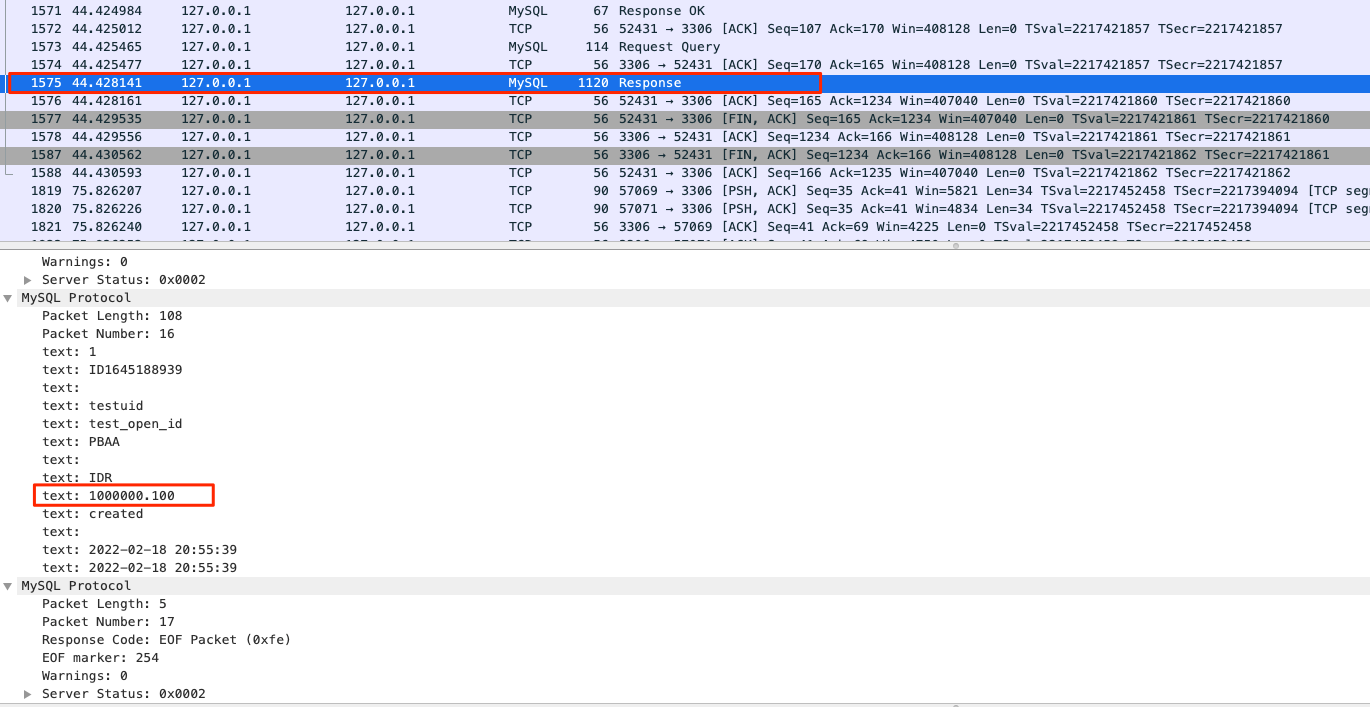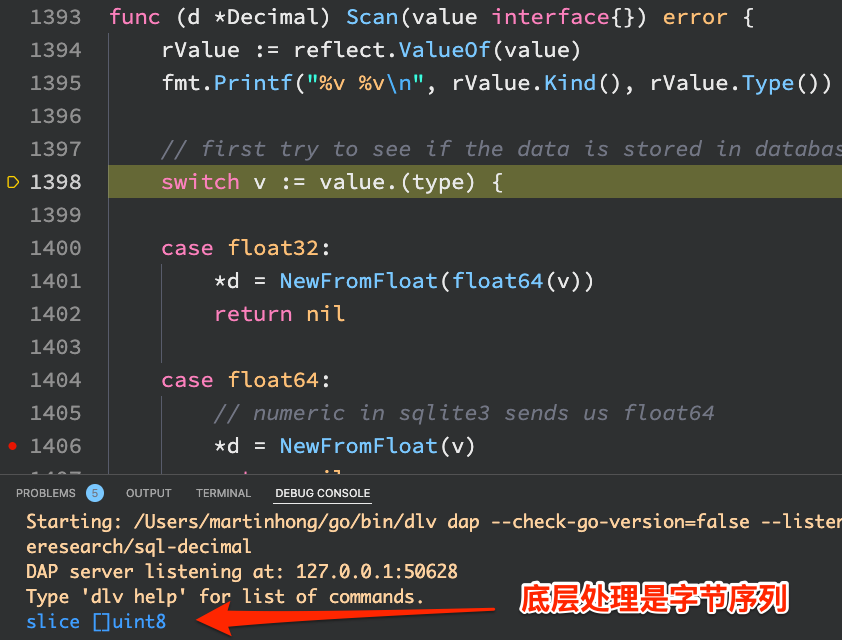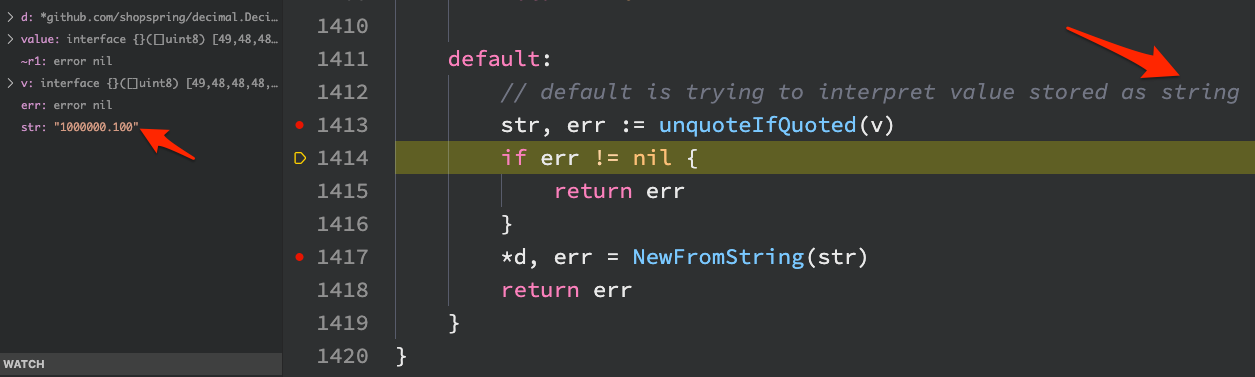In e-commerce or finance-related scenarios, data such as product prices involve the representation or calculation of decimals, and there is a risk of precision loss if you use the built-in floating-point types of programming languages. In the application area, the `decimal` type was created, and the MySQL database has built-in support for the `decimal` data type, while programming languages generally have standard libraries or third-party libraries that provide implementations of the `decimal` type. This article quickly shows how to implement a full link to read `decimal` type data without worrying about losing data accuracy.

## Database Tier - MySQL

At the MySQL level, the values of type `decimal` are represented in binary, and the general conversion process is as follows.

1. dividing the data to be stored into two according to the integer and fractional parts, e.g. `1234567890.1234`, into `1234567890` and `1234`.
2. for the integer part, divide it in groups of 9 bits of digits each, from the low bits to the high bits, e.g. `1234567890` would be divided into `1` and `234567890`.
3. using the shortest byte sequence to represent each grouped integer separately, `1` above being `0b00000001`, while `234567890` would correspond to `0x0D-FB-38-D2`.
4. for the fractional part, use a similar grouping (from the high bit to the low bit) treatment, i.e. 1234 is represented as `0x04D2`.
5. Finally, the highest bit is inverted to get `0x81 0D FB 38 D2 04 D2`, which means that 7 bytes are used to represent the number.

Bonus: If it is a decimal number, for example `-1234567890.1234`, just invert all the bits in step 5 above, that is `0x7E F2 04 C7 2D FB 2D`

### Summary

MySQL enables the representation of decimals with strict precision requirements by cleverly designed variable-length binary conversions.

## Network Transport Layer - MySQL

The decimal stored on MySQL’s underlying storage, after we know that it is binary, we also feel relieved about the accuracy issue of persistent storage, however, brings two more problems:

1. data after binary conversion, if the byte sequence to the client, the client obviously can not understand, and coupled with the conversion logic, it is clear that the MySQL server is required to do a reverse conversion from binary data to the real decimal.
2. how does MySQL ensure the security of the converted data transmitted in the database connection?

The answer is simple: plain text.

This can be confirmed by analyzing the packets transmitted by the MySQL connection, and the screenshot shows the decimal data returned by the MySQL server through Wireshark grabbing.### Summary

Because plain text is used to transfer the data, you don’t have to worry about the precision of decimals during the transfer.

## Application Layer - Golang

In my application, I used golang to develop the application, relying on the shopspring/decimal package to handle the decimal type, and it also implements the sql.Scanner interface, which means I can use it directly to deserialize data returned by database queries. For example, in my code.

 ``````1 2 3 4 5 6 7 8 9 `````` ``````// Order ... type Order struct { OrderNo string PurchaseAmount decimal.Decimal Status uint8 } order := new(Order) db.Where("order_no = ?", orderNo).First(order).Error ``````

Without any additional logic, `PurchaseAmount` is able to deserialize decimal type data exactly.

Nevertheless, I took a look at the implementation of the `Scanner` interface in the shopspring/decimal package to make sure it was indeed safe.

First, I added two lines of code at the source code to make it easier for me to confirm the type of the underlying data, and to confirm that it is a sequence of bytes before deserialization.After that, I traced the execution of the code and could see that the decimal package deserializes the data directly as a string.## Network Transport Layer - protobuf

Considering the risk of integer overflow and loss of floating point precision, I have also standardized on using string types in the protocol specification for external services.

 ``````1 2 3 4 5 `````` ``````message Order { string order_no = 1; string purchase_amount = 2; status int32 = 3; } ``````

## Summary

• MySQL uses variable-length binary representation of decimal-type data at the underlying level.
• MySQL uses plain text to represent decimal type data in network transfers.
• Golang programs use `shopspring/decimal` to handle decimal type data.
• `shopspring/decimal` uses scientific notation to represent decimal at the bottom, but this article will not expand on that.
• The application foreign service protocol uses strings to represent decimal type data.

## Think

• Using strings to represent decimal type data may introduce a higher number of bytes.